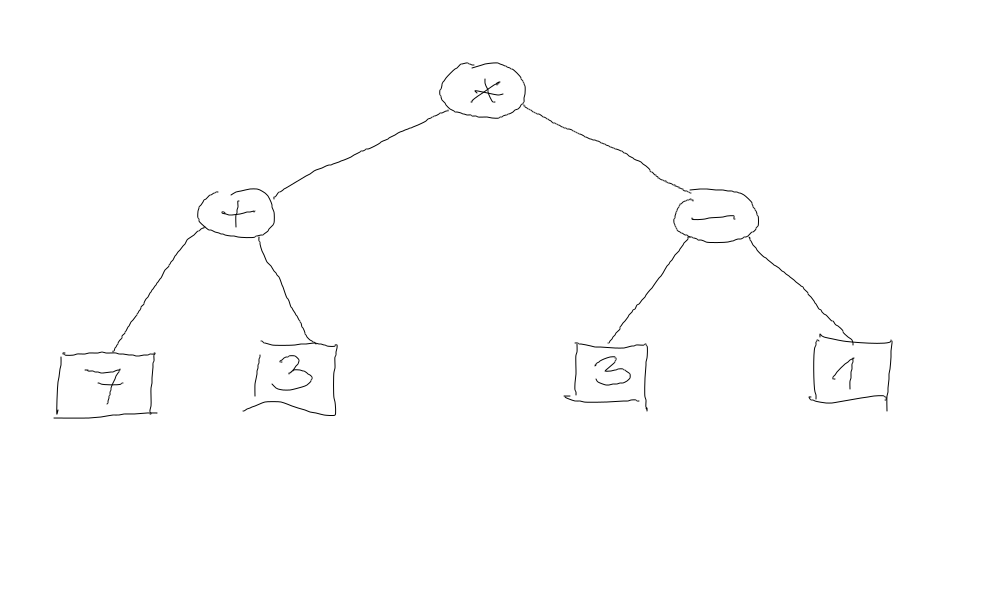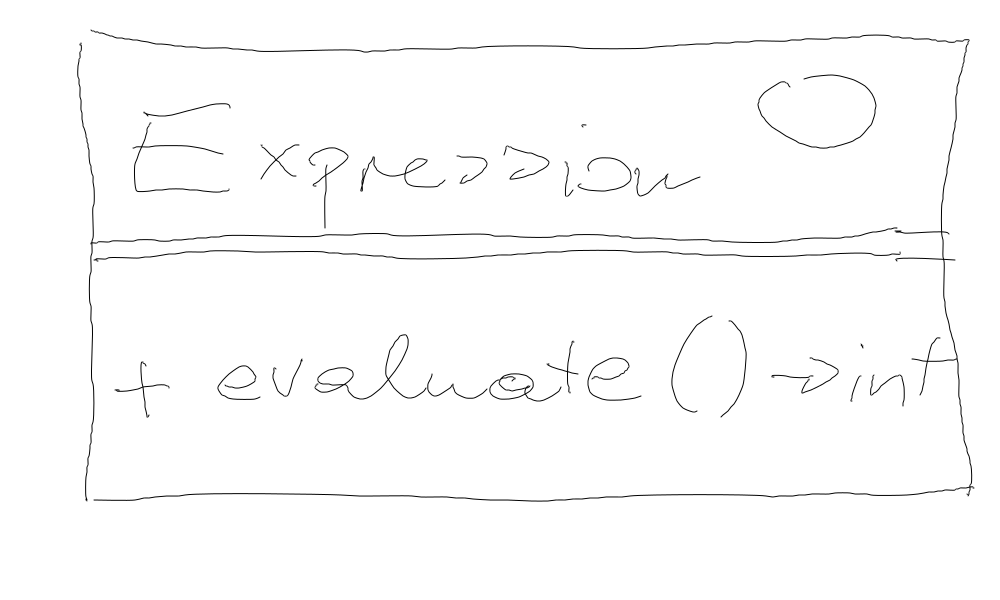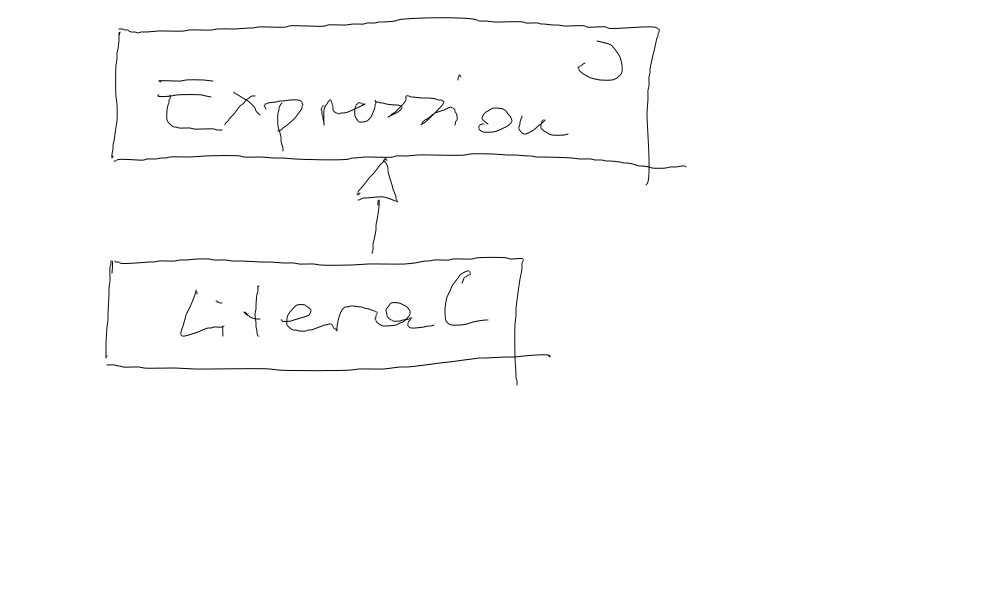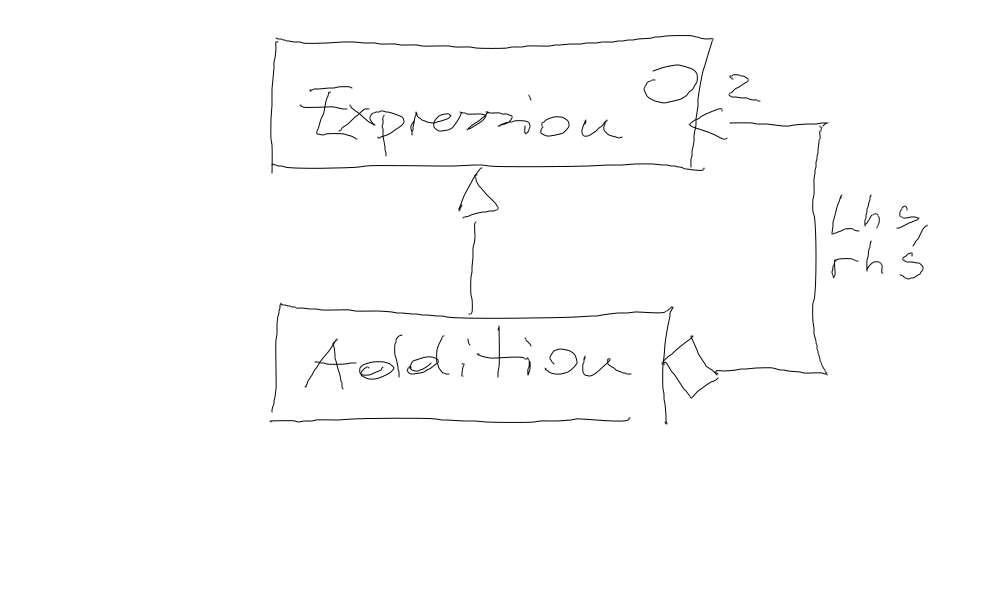# Exercise: Arithmetic Expression Interpreter¶

## Motivation¶

Create an Abstract Syntax Tree (AST), like compilers do (albeit much more sophisticated), to represent and evaluate expressions.

Type

Expression

Object structure

Literal

`42`Arithmetic expression tree

`(7+3)*(3-1)`## `Expression` Interface¶

For simplicity, all expressions evaluate to integer types.## Literals¶

In programming languages, a literal is a value that is written as-is, such as

```42
```

In itself, a literal does not make much sense. It is used in combinatin with operators to form larger expressions, such as ```42 + 5```. A literal is an expression, though, because it evaluates to a value.

 ```#include #include TEST(interpreter_suite, literal) { Literal le(42); Expression* e = ≤ // <--- Literal *is-a* Expression ASSERT_EQ(e->evaluate(), 42); // <--- ... and is evaluated as such } ```## Arithmetic (Binary) Expressions¶

• Binary arithmetic expressions come next: `+`, `-`, `*`, `/`

• Here’s a test, and a class diagram for `Addition`

 ```#include #include TEST(interpreter_suite, addition) { Literal lhs(1); Expression& e_lhs = lhs; Literal rhs(2); Expression& e_rhs = rhs; Addition add(e_lhs, e_rhs); // <--- adds two *Expression* values (not only Literals) Expression& e_add = add; // <--- Addition *is-a* Expression ASSERT_EQ(e_add.evaluate(), 3); // <--- ... and is evaluated as such } ```• `Subtraction`

```#include <gtest/gtest.h>

#include <expression.h>

TEST(interpreter_suite, subtraction)
{
Literal lhs(1);
Expression& e_lhs = lhs;

Literal rhs(2);
Expression& e_rhs = rhs;

Subtraction sub(e_lhs, e_rhs);
Expression& e_sub = sub;

ASSERT_EQ(e_sub.evaluate(), -1);
}
```
• `Multiplication`

```#include <gtest/gtest.h>

#include <expression.h>

TEST(interpreter_suite, multiplication)
{
Literal lhs(2);
Expression& e_lhs = lhs;

Literal rhs(3);
Expression& e_rhs = rhs;

Multiplication mul(e_lhs, e_rhs);
Expression& e_mul = mul;

ASSERT_EQ(e_mul.evaluate(), 6);
}
```
• `Division`

```#include <gtest/gtest.h>

#include <expression.h>

TEST(interpreter_suite, division)
{
Literal lhs(3);
Expression& e_lhs = lhs;

Literal rhs(2);
Expression& e_rhs = rhs;

Division div(e_lhs, e_rhs);
Expression& e_div = div;

ASSERT_EQ(e_div.evaluate(), 1);
}
```

## Check: Combined Arithmetic Expressions¶

`Literal`, and all of the arithemetic operators, are-a `Expression`. The arithemetic operators use-two `Expression` objects, so it should be possible to create expression trees like,

Test

Expression

Tree

```#include <gtest/gtest.h>

#include <expression.h>

TEST(interpreter_suite, combined_arith)
{
Literal l7(7);
Literal l3(3);
Literal l1(1);

Subtraction s_3_minus_1(l3, l1);

Multiplication total(a_7_plus_3, s_3_minus_1);

ASSERT_EQ(total.evaluate(), 20);
}
```

`(7+3)*(3-1) = 20`## And Variables?¶

Variables in programming languages live in a context - usually global or local scope (with their own lookup rules). Assignment to a variable drops a value into that variable’s context/scope.

For example, the following C function carries a local variable, `i`. Assignment to `i` write the assigned value into the memory location (the address) of `i`. The context of `i` is said to be the stack frame of the `function()` invocation.

```void function()
{
int i;
i = 42;
}
```

## No Uninitialized Variables!!¶

Lets say, our variable are always initialized - without ever assigning to them, they have a defined value: `0`.

```#include <gtest/gtest.h>

#include <expression.h>

TEST(interpreter_suite, variable_not_set)
{
Context context;              // <--- e.g. local or global scope (if we had functions)
Variable v(context, "i");     // <--- v lives in context, under the name "i"
ASSERT_EQ(v.evaluate(), 0);   // <--- v has never been assigned to -> default value 0
}
```

## Assigning To Variables¶

In the C analogy above, assigning to `i` write the assigned value into the variable’s context/scope. Additionally, we implement C’s “assignment has a value” semantics,

```int assignment_value;
int i;

assignment_value = i = 42;
```

I.e., the assignment `i = 42` is an expression whose value is `42`.

```#include <gtest/gtest.h>

#include <expression.h>

TEST(interpreter_suite, variable_assignment)
{
Context context;

Variable v(context, "i");
Literal l(42);
Assignment ass(v, l);               // <--- Assignment object created (but not yet evaluated)

ASSERT_EQ(ass.evaluate(), 42);      // <--- Assignment evaluation: "i = 42" has value 42 (as in C)
ASSERT_EQ(context.get("i"), 42);    // <--- variable has made it into context
ASSERT_EQ(v.evaluate(), 42);        // <--- variable now has a defined value
}
```

## Using Variables As Expressions¶

Obviously, in programming languages, variables can be used as expressions themselves.

```int i = 42;
int j = i;  // <--- i used as expression whose value is assigned to j
```
```#include <gtest/gtest.h>

#include <expression.h>

TEST(interpreter_suite, variable_used_as_expression)
{
Context context;

Variable v1(context, "i");
Literal l(42);
Assignment ass_v1(v1, l);
ass_v1.evaluate();             // <--- "i = 42" (not consuming assignment as expression)

Variable v2(context, "j");
Assignment ass_v2(v2, v1);
ass_v2.evaluate();             // <--- "i = j"

ASSERT_EQ(v2.evaluate(), 42);
}
```